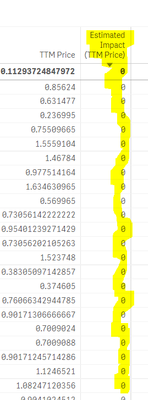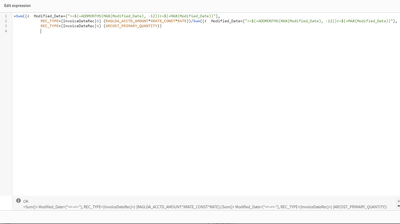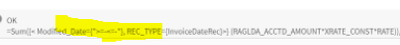Announcements
The way to achieve your own success is the willingness to help somebody else. Go for it!
cancel
Showing results for
Did you mean:Contributor III

## Formula Calculation Not being done

Hi All,

Greetings!

So this time I  am facing issue with the formula which is not giving the output, instead its just displaying 0.The formula that I am using here is as below:=(((Sum({<REC_TYPE={InvoiceDateRec}>} (RAGLDA_ACCTD_AMOUNT*XRATE_CONST*RATE))/num(SUM({<REC_TYPE={InvoiceDateRec}>} ARCOST_PRIMARY_QUANTITY),'#,##0.00'))
-
REC_TYPE={InvoiceDateRec}>} (ARCOST_PRIMARY_QUANTITY)))
*
num(SUM({<REC_TYPE={InvoiceDateRec}>} ARCOST_PRIMARY_QUANTITY))) /3

Overview of the formula is:

Calculation:
(Invoice Actual(CC)/Invoice Primary UOM Quantity)-TTM price *Invoice Primary UOM Quantity/3

(Above formula is in this same sequence)

Not sure what is going wrong here...but all the formulas that are combined and being used for this calculation  are being calculated properly and getting output also.

Say the 1st column 'TTM Price'(Please see Screenshot) is giving me the value, and formula that i have used for that is as below, and is also a part of the second column 'Estimated Impact (TTM Price)', as highlighted in the above formula.Akshaya

Labels (1)
• ### General Question

2 RepliesMVP

HI Akshaya,

Might be, due to date format

REC_TYPE={InvoiceDateRec}>} (ARCOST_PRIMARY_QUANTITY)))

If not, pls post the same file..  Modified_Date is date format or in text format?

Its blank here.Thanks & Regards, Mayil Vahanan R